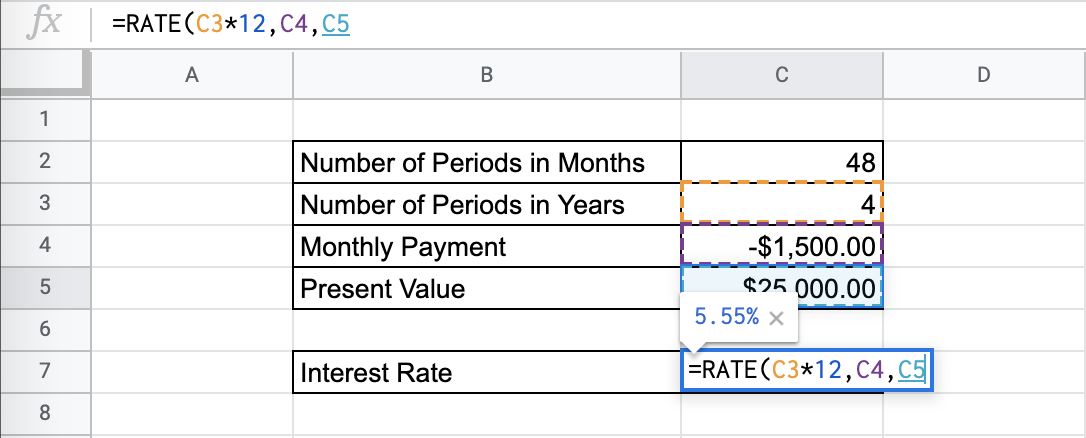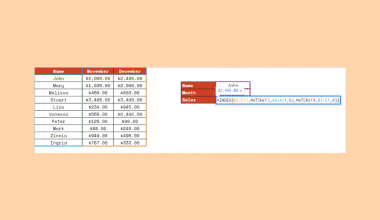# How to Use the RATE Function in Google Sheets

The RATE function in Google Sheets is used to calculate the interest rate of an annuity investment based on a range of constant-amount periodic payments and a constant interest rate.

This function is useful because it helps you find out the investment interest rate, given that you have the number of periodic payments and the constant payment value. It is an easy way to calculate it with the given information.

Let’s look at an example.

You want to choose what car loan to pursue, and you have been given the payment amount for each month over the next four years that you need in order to pay off the loan. You have the information about the cost of the investment, the monthly payment amount, and the number of periods that the payment will take. What is the underlying interest rate?

The `RATE` function takes in all these inputs in order to calculate the constant interest rate.

## The Anatomy of the RATE Function in Google Sheets

The syntax of the `RATE` function is as follows::

`=RATE(number_of_periods, payment_per_period, present_value, [future_value, end_or_beginning, rate_guess])`

Let’s have a look at each part of the function to understand what is going on here:

• `=` is the equals sign that starts off any function in Google Sheets.
• `RATE` is the name of our function.
• `number_of_periods` is the number of payments to be made.
• `payment_per_period` is the amount per period to be paid.
• `present_value` is the current value of the annuity.
• `future_value` is the optional attribute that is the future value remaining after the final payment has been made.
• `end_or_beginning` is an optional attribute that sets whether the payments are due at the end (0) or the beginning (1) of each period. If left blank, it will be 0 by default.
• `rate_guess` is an optional attribute that is your estimate for the interest rate, if you have any good idea of what it may be. If left blank, it will be 0.1 by default.

## A Real Example of Using the RATE Function

Let’s look at the example below to see how to use `RATE` function in Google Sheets.

#### Calculating the Interest Rate in Google Sheets

This is a simple problem. We want to find the interest rate for a 4-year car loan.

``

The function takes three arguments, with three optional arguments. So in the equation, it will look like:

`=RATE(C3*12,C4,C5,0,0,0)`

As mentioned above, because we are looking for an annual percentage rate, we need to keep our payment periods and rates consistent. That is why, as you can see in the example, the number of periods, in years, is multiplied by 12.

As a result, we get 5.55%.

This simple problem can be practiced to perfection. Use the link below to get a copy of this problem set:

## How to Use the RATE Function in Google Sheets

In this section, we will show you a step-by-step process on how to use the `RATE` function in Google Sheets.

In this problem, we will be calculating the interest rate in a loan.

1. To begin, click on a cell to make active, which you would like to display the RATE. For this guide, the RATE will be in Cell C7.1. Next, type the equal sign ‘=’ to start writing the function. Follow this with “RATE” or “rate” – Google Sheets functions are not case sensitive, so either is fine.1. The auto-suggest box will create a drop-down menu. Select the RATE function by clicking it. It is the first to pop up on the list, but take care to choose the correct function.1. After the opening bracket ‘(‘, you will add the rate attribute. Note that since we are given monthly payments and an annual percentage rate, we need to multiply the rate by 12, as seen in the formula below.1. Next, we enter the monthly payment amount.1. Next, we enter the present value of the investment.1. Next, there is an option to put a future value. You can leave it blank, or leave it at 0.1. Next, we have to choose if the payment will be at the end or the beginning of the payment period. You can choose 1 if you pay at the beginning of the month, or 0 if you pay at the end of the month – which is the default setting. In this problem, we choose 0.1. Next, there is the optional attribute of guessing the rate. You can leave it at 0 or blank. We’re almost done! You should even see a preview for the end result.1. Hit Enter and you will find the annual interest rate.Given a practical problem where you should completely solve for the correct annual interest rate, use the `RATE` function to find and study the interest rate for your important financial decision-making.

And there you have it – you can now use the `RATE` function in Google Sheets together with the other numerous Google Sheets formulas to create even more effective formulas.Our goal this year is to create lots of rich, bite-sized tutorials for Google Sheets users like you. If you liked this one, you'll love what we are working on! Readers receive ✨ early access ✨ to new content. There will be no spam and you can unsubscribe at any time.

##### You May Also Like## How To Use INDEX and MATCH Together in Google Sheets

The INDEX and MATCH together in Google Sheets come useful if you want to perform lookups. Using the…## How to Use COUNTIF Function in Google Sheets

The COUNTIF function in Google Sheets is useful if you want to count the number of times a…## How to Use the MMULT Function in Google Sheets

The MMULT function in Google Sheets is short for “matrix multiplication function” and it automates row-to-column multiplication for…## How to Use STDEV Function in Google Sheets

The STDEV Function in Google Sheets is useful if you want to find out the standard deviation from…